Published on Dec 25,2018
99.6K Views
Email Post

## MapReduce Tutorial: Introduction

In this MapReduce Tutorial blog, I am going to introduce you to MapReduce, which is one of the core building blocks of processing in Hadoop framework. Before moving ahead, I would suggest you to get familiar with HDFS concepts which I have covered in my previous HDFS tutorial blog. This will help you to understand the MapReduce concepts quickly and easily.

Google released a paper on MapReduce technology in December, 2004. This became the genesis of the Hadoop Processing Model. So, MapReduce is a programming model that allows us to perform parallel and distributed processing on huge data sets. The topics that I have covered in this MapReduce tutorial blog are as follows:

## MapReduce Tutorial: Traditional Way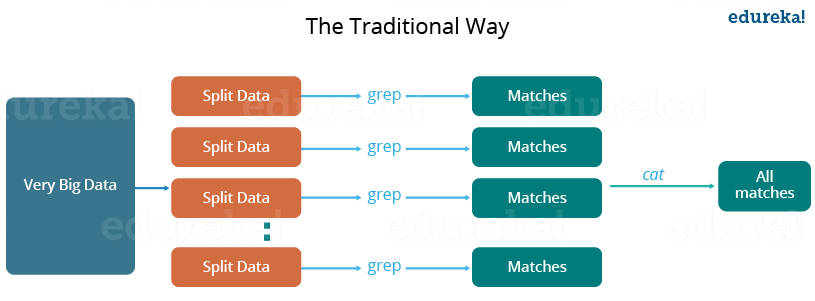Let us understand, when the MapReduce framework was not there, how parallel and distributed processing used to happen in a traditional way. So, let us take an example where I have a weather log containing the daily average temperature of the years from 2000 to 2015. Here, I want to calculate the day having the highest temperature in each year.

So, just like in the traditional way, I will split the data into smaller parts or blocks and store them in different machines. Then, I will find the highest temperature in each part stored in the corresponding machine. At last, I will combine the results received from each of the machines to have the final output. Let us look at the challenges associated with this traditional approach:

1. Critical path problem: It is the amount of time taken to finish the job without delaying the next milestone or actual completion date. So, if, any of the machines delays the job, the whole work gets delayed.
2. Reliability problem: What if, any of the machines which is working with a part of data fails? The management of this failover becomes a challenge.
3. Equal split issue: How will I divide the data into smaller chunks so that each machine gets even part of data to work with. In other words, how to equally divide the data such that no individual machine is overloaded or under utilized.
4. Single split may fail: If any of the machine fails to provide the output, I will not be able to calculate the result. So, there should be a mechanism to ensure this fault tolerance capability of the system.
5. Aggregation of result: There should be a mechanism to aggregate the result generated by each of the machines to produce the final output.

These are the issues which I will have to take care individually while performing parallel processing of huge data sets when using traditional approaches.

To overcome these issues, we have the MapReduce framework which allows us to perform such parallel computations without bothering about the issues like reliability, fault tolerance etc. Therefore, MapReduce gives you the flexibility to write code logic without caring about the design issues of the system.

## MapReduce Tutorial: What is MapReduce?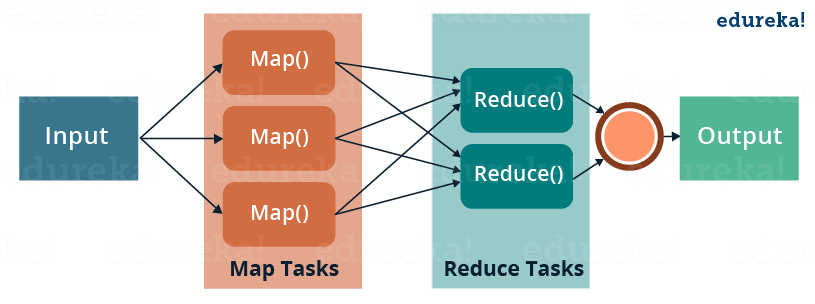MapReduce is a programming framework that allows us to perform distributed and parallel processing on large data sets in a distributed environment.

• MapReduce consists of two distinct tasks – Map and Reduce.
• As the name MapReduce suggests, reducer phase takes place after mapper phase has been completed.
• So, the first is the map job, where a block of data is read and processed to produce key-value pairs as intermediate outputs.
• The output of a Mapper or map job (key-value pairs) is input to the Reducer.
• The reducer receives the key-value pair from multiple map jobs.
• Then, the reducer aggregates those intermediate data tuples (intermediate key-value pair) into a smaller set of tuples or key-value pairs which is the final output.

Meanwhile, you may go through this MapReduce Tutorial video where our expert from Hadoop online training has discussed all the concepts related to MapReduce has been clearly explained using examples:

## MapReduce Tutorial: A Word Count Example of MapReduce

Let us understand, how a MapReduce works by taking an example where I have a text file called example.txt whose contents are as follows:

Dear, Bear, River, Car, Car, River, Deer, Car and Bear

Now, suppose, we have to perform a word count on the sample.txt using MapReduce. So, we will be finding the unique words and the number of occurrences of those unique words.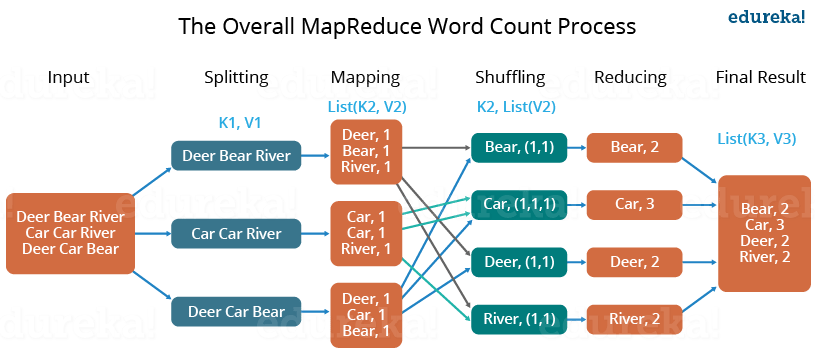• First, we divide the input in three splits as shown in the figure. This will distribute the work among all the map nodes.
• Then, we tokenize the words in each of the mapper and give a hardcoded value (1) to each of the tokens or words. The rationale behind giving a hardcoded value equal to 1 is that every word, in itself, will occur once.
• Now, a list of key-value pair will be created where the key is nothing but the individual words and value is one. So, for the first line (Dear Bear River) we have 3 key-value pairs – Dear, 1; Bear, 1; River, 1. The mapping process remains the same on all the nodes.
• After mapper phase, a partition process takes place where sorting and shuffling happens so that all the tuples with the same key are sent to the corresponding reducer.
• So, after the sorting and shuffling phase, each reducer will have a unique key and a list of values corresponding to that very key. For example, Bear, [1,1]; Car, [1,1,1].., etc.
• Now, each Reducer counts the values which are present in that list of values. As shown in the figure, reducer gets a list of values which is [1,1] for the key Bear. Then, it counts the number of ones in the very list and gives the final output as – Bear, 2.
• Finally, all the output key/value pairs are then collected and written in the output file.

## MapReduce Tutorial: Advantages of MapReduce

The two biggest advantages of MapReduce are:

### 1. Parallel Processing:

In MapReduce, we are dividing the job among multiple nodes and each node works with a part of the job simultaneously. So, MapReduce is based on Divide and Conquer paradigm which helps us to process the data using different machines. As the data is processed by multiple machine instead of a single machine in parallel, the time taken to process the data gets reduced by a tremendous amount as shown in the figure below (2).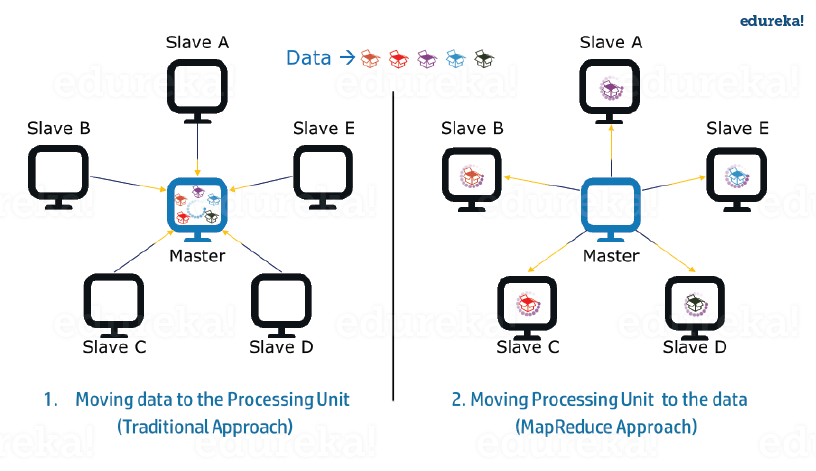Fig.: Traditional Way Vs. MapReduce Way – MapReduce Tutorial

### 2. Data Locality:

Instead of moving data to the processing unit, we are moving processing unit to the data in the MapReduce Framework.  In the traditional system, we used to bring data to the processing unit and process it. But, as the data grew and became very huge, bringing this huge amount of data to the processing unit posed following issues:

• Moving huge data to processing is costly and deteriorates the network performance.
• Processing takes time as the data is processed by a single unit which becomes the bottleneck.
• Master node can get over-burdened and may fail.

Now, MapReduce allows us to overcome above issues by bringing the processing unit to the data. So, as you can see in the above image that the data is distributed among multiple nodes where each node processes the part of the data residing on it. This allows us to have the following advantages:

• It is very cost effective to move processing unit to the data.
• The processing time is reduced as all the nodes are working with their part of the data in parallel.
• Every node gets a part of the data to process and therefore, there is no chance of a node getting overburdened.Trending Courses in this category

#### Big Data Hadoop Certification Training

5 (57650)
145k Learners Enrolled
Best Price 17,995 19,995

## MapReduce Tutorial: MapReduce Example Program

Before jumping into the details, let us have a glance at a MapReduce example program to have a basic idea about how things work in a MapReduce environment practically. I have taken the same word count example where I have to find out the number of occurrences of each word. And Don’t worry guys, if you don’t understand the code when you look at it for the first time, just bear with me while I walk you through each part of the MapReduce code.

### Source code:

```
package co.edureka.mapreduce;
import java.io.IOException;
import java.util.StringTokenizer;

public class WordCount
{
public static class Map extends Mapper<LongWritable,Text,Text,IntWritable> {
public void map(LongWritable key, Text value,Context context) throws IOException,InterruptedException{
String line = value.toString();
StringTokenizer tokenizer = new StringTokenizer(line);
while (tokenizer.hasMoreTokens()) {
value.set(tokenizer.nextToken());
context.write(value, new IntWritable(1));
}
}
}

public static class Reduce extends Reducer<Text,IntWritable,Text,IntWritable> {
public void reduce(Text key, Iterable<IntWritable> values,Context context) throws IOException,InterruptedException {
int sum=0;
for(IntWritable x: values)
{
sum+=x.get();
}
context.write(key, new IntWritable(sum));
}
}

public static void main(String[] args) throws Exception {

Configuration conf= new Configuration();
Job job = new Job(conf,"My Word Count Program");
job.setJarByClass(WordCount.class);
job.setMapperClass(Map.class);
job.setReducerClass(Reduce.class);
job.setOutputKeyClass(Text.class);
job.setOutputValueClass(IntWritable.class);
job.setInputFormatClass(TextInputFormat.class);
job.setOutputFormatClass(TextOutputFormat.class);
Path outputPath = new Path(args);
//Configuring the input/output path from the filesystem into the job
FileOutputFormat.setOutputPath(job, new Path(args));
//deleting the output path automatically from hdfs so that we don't have to delete it explicitly
outputPath.getFileSystem(conf).delete(outputPath);
//exiting the job only if the flag value becomes false
System.exit(job.waitForCompletion(true) ? 0 : 1);
}
}

```

## MapReduce Tutorial: Explanation of MapReduce Program

The entire MapReduce program can be fundamentally divided into three parts:

• Mapper Phase Code
• Reducer Phase Code
• Driver Code

We will understand the code for each of these three parts sequentially.

## Mapper code:

```
public static class Map extends Mapper<LongWritable,Text,Text,IntWritable> {

public void map(LongWritable key, Text value, Context context) throws IOException,InterruptedException {

String line = value.toString();
StringTokenizer tokenizer = new StringTokenizer(line);
while (tokenizer.hasMoreTokens()) {
value.set(tokenizer.nextToken());
context.write(value, new IntWritable(1));
}

```
• We have created a class Map that extends the class Mapper which is already defined in the MapReduce Framework.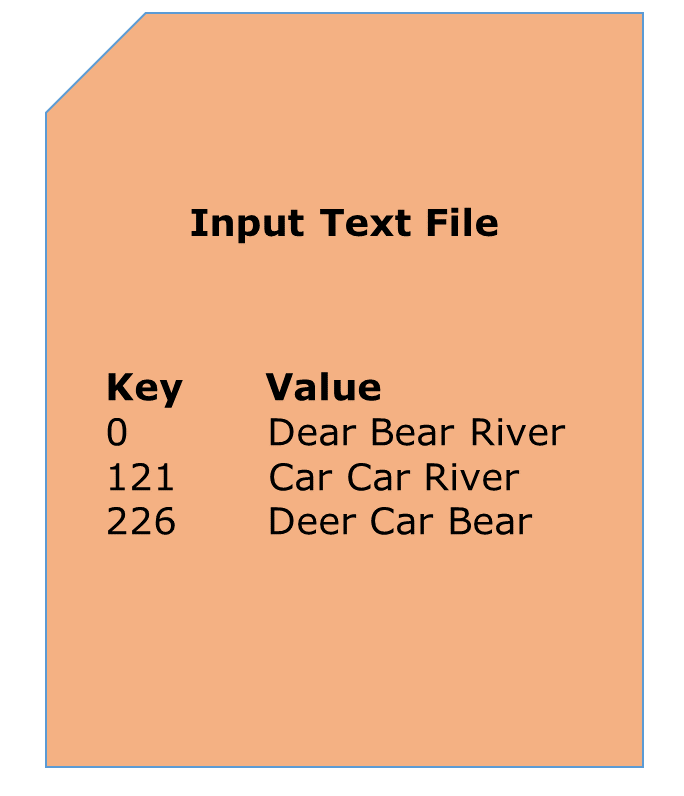• We define the data types of input and output key/value pair after the class declaration using angle brackets.
• Both the input and output of the Mapper is a key/value pair.
• Input:
• The key is nothing but the offset of each line in the text file: LongWritable
• The value is each individual line (as shown in the figure at the right): Text
• Output:
• The key is the tokenized words: Text
• We have the hardcoded value in our case which is 1: IntWritable
• Example – Dear 1, Bear 1, etc.
• We have written a java code where we have tokenized each word and assigned them a hardcoded value equal to 1.

## Reducer Code:

```
public static class Reduce extends Reducer<Text,IntWritable,Text,IntWritable> {

public void reduce(Text key, Iterable<IntWritable> values,Context context)
throws IOException,InterruptedException {

int sum=0;
for(IntWritable x: values)
{
sum+=x.get();
}
context.write(key, new IntWritable(sum));
}
}

```
• We have created a class Reduce which extends class Reducer like that of Mapper.
• We define the data types of input and output key/value pair after the class declaration using angle brackets as done for Mapper.
• Both the input and the output of the Reducer is a key-value pair.
• Input:
• The key nothing but those unique words which have been generated after the sorting and shuffling phase: Text
• The value is a list of integers corresponding to each key: IntWritable
• Example – Bear, [1, 1], etc.
• Output:
• The key is all the unique words present in the input text file: Text
• The value is the number of occurrences of each of the unique words: IntWritable
• Example – Bear, 2; Car, 3, etc.
• We have aggregated the values present in each of the list corresponding to each key and produced the final answer.
• In general, a single reducer is created for each of the unique words, but, you can specify the number of reducer in mapred-site.xml.

## Driver Code:

```
Configuration conf= new Configuration();
Job job = new Job(conf,"My Word Count Program");
job.setJarByClass(WordCount.class);
job.setMapperClass(Map.class);
job.setReducerClass(Reduce.class);
job.setOutputKeyClass(Text.class);

job.setOutputValueClass(IntWritable.class);
job.setInputFormatClass(TextInputFormat.class);
job.setOutputFormatClass(TextOutputFormat.class);
Path outputPath = new Path(args);

//Configuring the input/output path from the filesystem into the job
FileOutputFormat.setOutputPath(job, new Path(args));

```
• In the driver class, we set the configuration of our MapReduce job to run in Hadoop.
• We specify the name of the job , the data type of input/output of the mapper and reducer.
• We also specify the names of the mapper and reducer classes.
• The path of the input and output folder is also specified.
• The method setInputFormatClass () is used for specifying that how a Mapper will read the input data or what will be the unit of work. Here, we have chosen TextInputFormat so that single line is read by the mapper at a time from the input text file.
• The main () method is the entry point for the driver. In this method, we instantiate a new Configuration object for the job.

## Run the MapReduce code:

The command for running a MapReduce code is:

Now, you guys have a basic understanding of MapReduce framework. You would have realized how the MapReduce framework facilitates us to write code to process huge data present in the HDFS. There has been significant changes in the MapReduce framework in Hadoop 2.x as compared to Hadoop 1.x. These changes will be discussed in the next blog of this MapReduce tutorial series. I will share a downloadable comprehensive guide which explains each part of the MapReduce program in that very blog.

Now that you have understood what is MapReduce and its advantages, check out the Hadoop training by Edureka, a trusted online learning company with a network of more than 250,000 satisfied learners spread across the globe. The Edureka Big Data Hadoop Certification Training course helps learners become expert in HDFS, Yarn, MapReduce, Pig, Hive, HBase, Oozie, Flume and Sqoop using real-time use cases on Retail, Social Media, Aviation, Tourism, Finance domain.

Got a question for us? Please mention it in the comments section and we will get back to you.